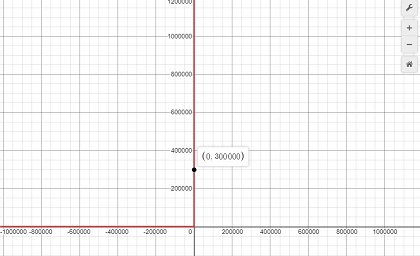# The present value of a building in the downtown area is given by the function p(t) = 300000e^...

## Question:

The present value of a building in the downtown area is given by the function {eq}p(t) = 300000e^{(-0.09t+ \frac{t}{2}}) {/eq} for {eq}0 \leq t \leq 10 {/eq}. Find the optimal present value of the building. (Hint: Use a graphing utility to graph the function, {eq}P(t) {/eq}, and find the value of {eq}t_0 {/eq} that gives a point on the graph, {eq}(t_0, P(t_0)) {/eq}, where the slope of the tangent line is {eq}0 {/eq}.

## Maxima/Minima of a Function:

Given a function f(x), if it attains a maximum or minimum at a point a, then {eq}\frac{df}{dx} |_{x=a} =0 {/eq}

To know whether the point gives a maximum or a minimum, we can apply the second derivative test at that point. If the second derivative at that point is negative, then the point corresponds to a maximum. If the second derivative at the point is positive, then it corresponds to a minimum.

The graph of the given function looks like below :It can be seen that the value keep increasing exponentially. Hence, in the given range of t, there is no point where the slope of the tangent to the graph becomes zero. The optimal present value of the building therefore is at t=10 i.e.,

{eq}p(10) = 300000e^{-0.9 + 5} \\ p(10) = 18102086.28 {/eq}